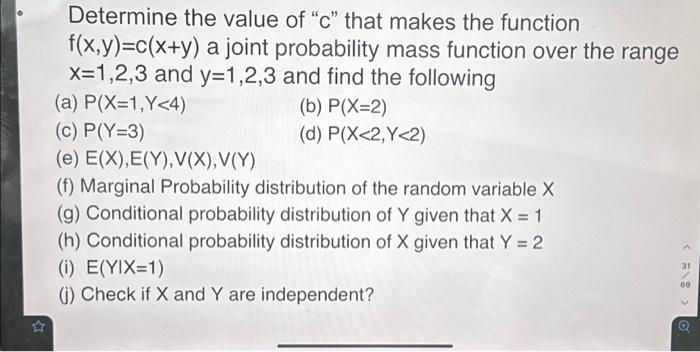Home / Expert Answers / Statistics and Probability / determine-the-value-of-34-c-34-that-makes-the-function-f-x-y-c-x-y-a-joint-probability-mass-function-pa465

# (Solved): Determine the value of "c" that makes the function f(x,y)=c(x+y) a joint probability mass function o ...

Determine the value of "c" that makes the function f(x,y)=c(x+y) a joint probability mass function over the range x=1,2,3 and y=1,2,3 and find the following (a) P(X=1,Y<4) (c) P(Y=3) (e) E(X),E(Y), V(X), V(Y) (f) Marginal Probability distribution of the random variable X (g) Conditional probability distribution of Y given that X = 1 (h) Conditional probability distribution of X given that Y = 2 (i) E(YIX=1) (j) Check if X and Y are independent? (b) P(X=2) (d) P(X<2,Y<2) < 31 69Determine the value of "c" that makes the function a joint probability mass function over the range and and find the following (a) (b) (c) (d) (e) (f) Marginal Probability distribution of the random variable (g) Conditional probability distribution of given that (h) Conditional probability distribution of given that (i) (j) Check if and are independent?

We have an Answer from Expert